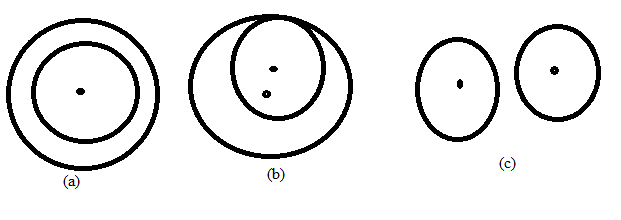Chapter 6.3, Problem 25EElementary Geometry For College St...

7th Edition
Alexander + 2 others
ISBN: 9781337614085

Solutions

Chapter
SectionElementary Geometry For College St...

7th Edition
Alexander + 2 others
ISBN: 9781337614085
Textbook Problem

For the two circles in figures (a), (b), and (c), find the total number of common tangents (internal and external).To determine

(a)

To find:

To find the total number of common tangents (internal and external).

Explanation

Consider the following figure,

To determine

(b)

To find:

To find the total number of common tangents (internal and external).

To determine

(c)

To find:

To find the total number of common tangents (internal and external).

Still sussing out bartleby?

Check out a sample textbook solution.

See a sample solution

The Solution to Your Study Problems

Bartleby provides explanations to thousands of textbook problems written by our experts, many with advanced degrees!

Get Started

Change 24 ft to yards.

Elementary Technical Mathematics

Graph the following functions. 3.

Mathematical Applications for the Management, Life, and Social Sciences

Find the length of the curve. 1. y = 4 (x 1)3/2, 1 x 4

Single Variable Calculus: Early Transcendentals

For y = 3x + tan x, y = _____. a) 3 + sec x b) 3x sec2 x + 3 tan x c) 3 + sec2 x d) x cos x + sin x

Study Guide for Stewart's Single Variable Calculus: Early Transcendentals, 8th